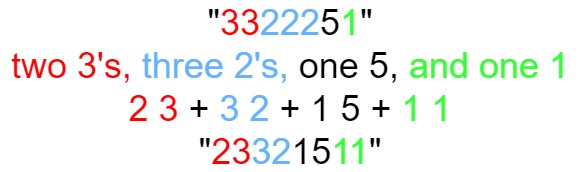38. Count and Say

The count-and-say sequence is a sequence of digit strings defined by the recursive formula:

• `countAndSay(1) = "1"`
• `countAndSay(n)` is the way you would "say" the digit string from `countAndSay(n-1)`, which is then converted into a different digit string.

To determine how you "say" a digit string, split it into the minimal number of groups so that each group is a contiguous section all of the same character. Then for each group, say the number of characters, then say the character. To convert the saying into a digit string, replace the counts with a number and concatenate every saying.

For example, the saying and conversion for digit string `"3322251"`:Given a positive integer `n`, return the `nth` term of the count-and-say sequence.

Example 1:

```Input: n = 1
Output: "1"
Explanation: This is the base case.
```

Example 2:

```Input: n = 4
Output: "1211"
Explanation:
countAndSay(1) = "1"
countAndSay(2) = say "1" = one 1 = "11"
countAndSay(3) = say "11" = two 1's = "21"
countAndSay(4) = say "21" = one 2 + one 1 = "12" + "11" = "1211"
```

Constraints:

• `1 <= n <= 30`

38. Count and Say
``````struct Solution;

struct Pair {
digit: char,
count: usize,
}

impl Solution {
fn next(nums: String) -> String {
let mut prev: Option<Pair> = None;
let mut s = String::from("");
for c in nums.chars() {
if let Some(prev_pair) = prev {
if prev_pair.digit == c {
prev = Some(Pair {
digit: c,
count: prev_pair.count + 1,
});
} else {
s.push_str(&prev_pair.count.to_string());
s.push_str(&prev_pair.digit.to_string());
prev = Some(Pair { digit: c, count: 1 });
}
} else {
prev = Some(Pair { digit: c, count: 1 });
}
}
if let Some(prev_pair) = prev {
s.push_str(&prev_pair.count.to_string());
s.push_str(&prev_pair.digit.to_string());
}
s
}

fn count_and_say(n: i32) -> String {
match n {
1 => String::from("1"),
2..=30 => Self::next(Solution::count_and_say(n - 1)),
_ => String::from(""),
}
}
}

#[test]
fn test() {
assert_eq!(Solution::count_and_say(4), String::from("1211"));
assert_eq!(Solution::count_and_say(1), String::from("1"));
}
``````